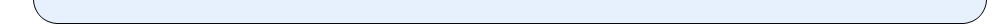•••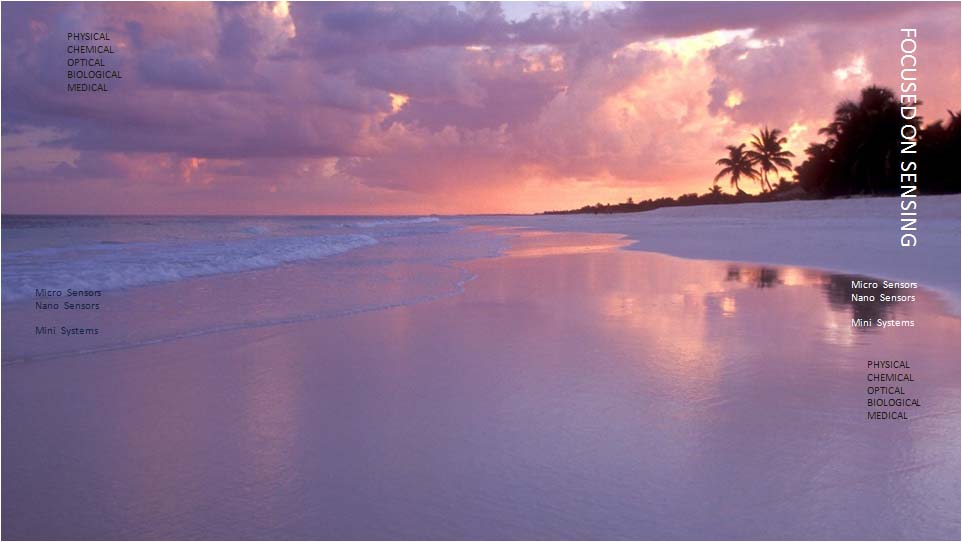••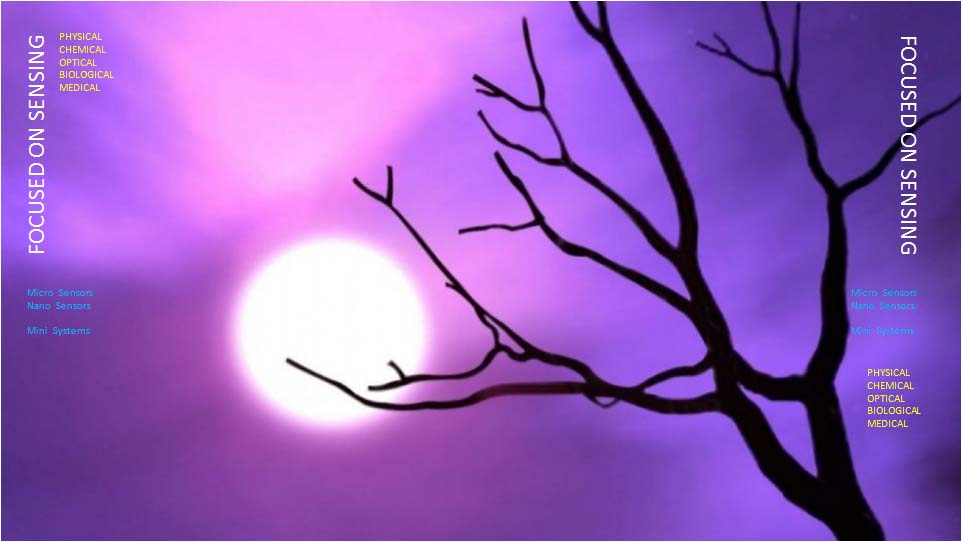•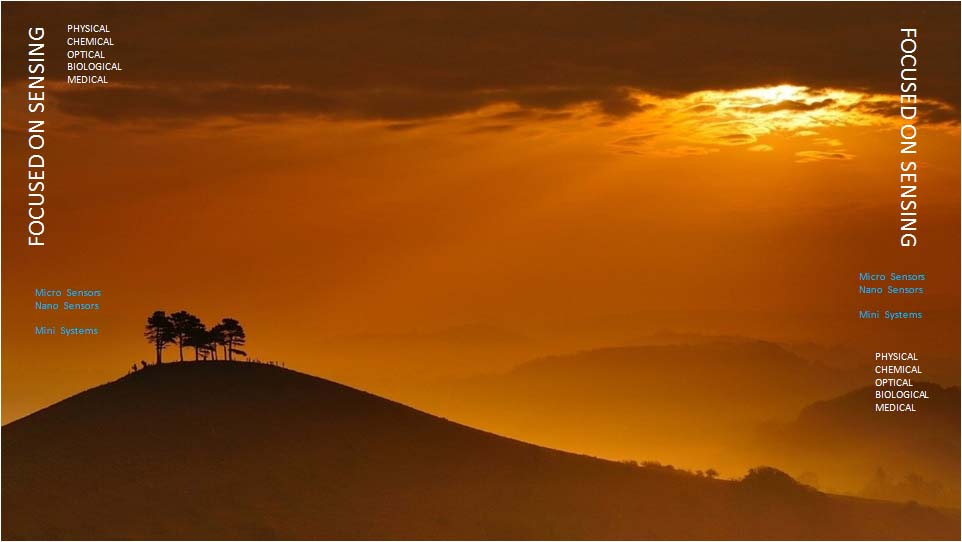•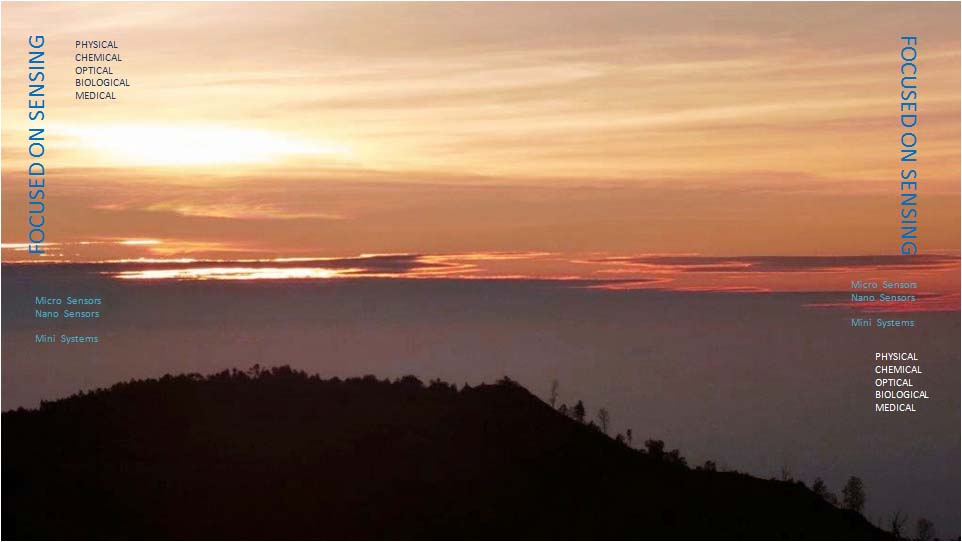•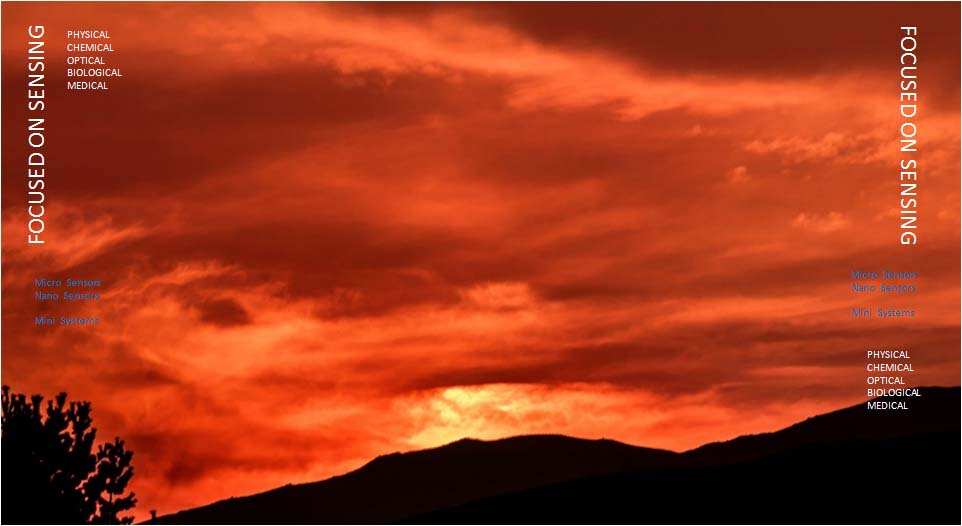•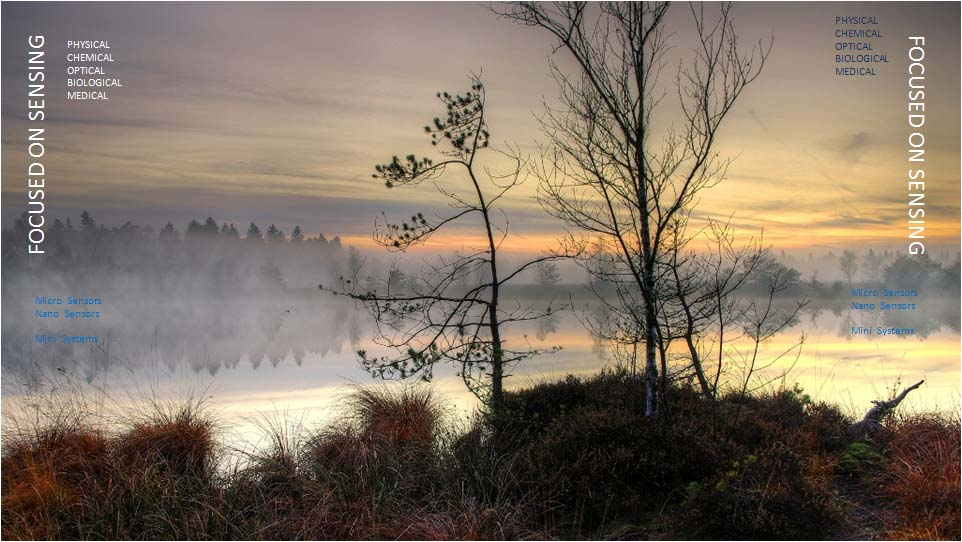•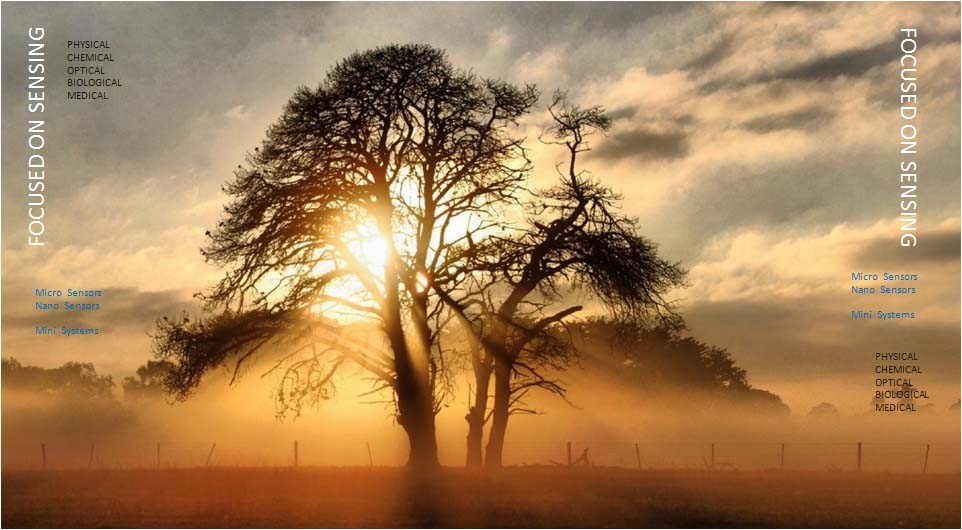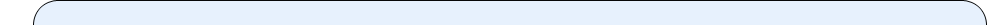|   Sensor Development Software   |

### Calibration Test Software

This software is used to extract basic calibration parameters of a sensor. The output of the sensor is recorded for a series of precisely correct inputs over the input range of the sensor. A regression polynomial is fitted into the IO data. If the sensor behavior is linear, the fitted curve is straight line, it yields the bias and scale factor of the sensor. Otherwise, a higher degree polynomial is selected with its corresponding coefficients. If the sensor behavior is stable, the regression analysis yields the same set of numbers. The sensor tests are repeated at different soaking temperatures. This type of testing has become quite routine with the advent of powerful spreadsheets and their automated data storage feature. No dedicated software is required.

### Test Data Storage & Archiving

Generally, before a system is fielded in actual use, all of its sub-modules have to go through a series of tests. Such testing produces a large volume of data. An organized software tool is employed to handle the data, and to correlate the data with processing source materials and equipment used. This gives us full tractability, and helps us perform improvements in future.
The system development software tracks and archives all tests and the resulting data. For example, the development software for AXLGYRO keeps track of the following test sequences and their data:

### Accelerometer Performance

The accelerometer parameters are measured on the unit under test at standard laboratory condition.

### Input Range

The input minimum range is measured. A +/- 1 g test is performed, and a range for the instrument is computed.

### Threshold

The accelerometer g threshold is measured with both the plus and minus input conditions.

### Warm-up Time

The turn on bias stabilization time is measured with a minimum off time of 4 hours.

### Scale Factor Testing Linearity

The scale factor linearity and asymmetry are measured over +/- 1 1 g with minimum of 12 position. The difference for the 1 g values is expressed in %.

### Short Term Stability

The rms deviation of the scale factor about the mean of several measurements over a 2- hour period is measured.

### Long Term Stability

Provide values and dates on which the scale factor measurements are taken over the test period.

### Repeatability

The rms deviation of the scale factor from the mean of 10 measurements taken with power recycling. The scale factor repeatability is measured across a minimum of a 4 hour power off condition. The device is maintained stable for both the pre and post turn on measurement.

### Temperature Sensitivity

The temperature sensitivity of the scale factor is measured over temperature of 30 *C. The scale factor is measured after stabilizing the temperature at every test set point 5 degrees apart.

### Bias Short Term Stability

The rms deviation of the bias from its mean value is measured over a period of 1 hour

### Bias Noise

The spectral noise is measured over the frequency range of 10 Hz to .1 Hz. The analog signal output of the accelerometer is sampled at a 100 Hz for a period 100 seconds.

### Repeatability

The rms deviation of the bias from the mean of 10 measurements is taken with power recycling.

### Temperature Sensitivity

The temperature sensitivity of the bias is measured over temperature of 30 *C. The bias is measured after stabilizing the temperature at every test set point 5 degrees apart.

### Vibration Sensitivity

The vibration sensitivity of the bias is measured using a sine sweep from 10 to 2000 Hz at 0.5 , 1, 4 g’s peak-to-peak.

### Input Axis (IA) Misalignment Short Term Stability

The rms deviation of the IA misalignment from the mean of several measurements taken over a 2-hour period.

### Input Axis (IA) Misalignment Long Term Stability

Provide values and dates on which the IA misalignment measurements are taken over the test period.

### Repeatability

The rms deviation of the IA misalignment from the mean of 10 measurements taken with power recycling.

### Frequency Response

The frequency response of the accelerometer servo loop is measured both for amplitude and phase from DC to 2000 Hz.

### Gyro Performance

Scale factor, bias and IA Misalignment tests similar to those performed for accelerometer are duplicated for the gyro. Waddan Systems uses a test station that combines a rate table and a dividing head. So both gyro and accelerometer can be tested simultaneously.

•